#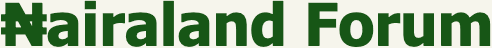Welcome, Guest: Join Nairaland / LOGIN! / Trending / Recent / New
Stats: 2,863,154 members, 6,869,461 topics. Date: Wednesday, 18 May 2022 at 08:29 PM

## Are You Good At Maths? Solve This - Education - Nairaland

 Are You Good At Maths? Solve This by zinoad: 10:47pm On Mar 06, 2015 Are you good at maths? solve this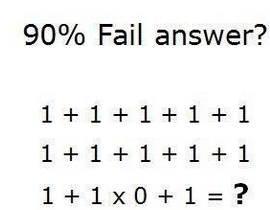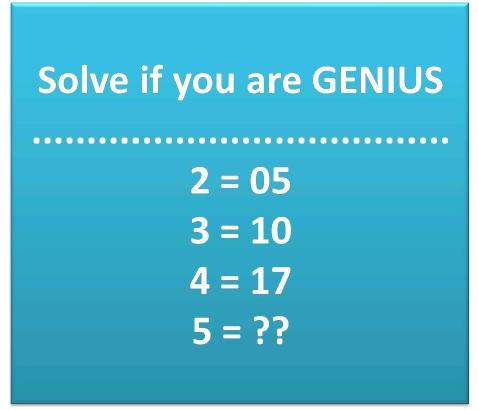Re: Are You Good At Maths? Solve This by simplemach(m): 10:53pm On Mar 06, 2015 Oga na 12 Re: Are You Good At Maths? Solve This by html14java(f): 11:01pm On Mar 06, 2015 12 Re: Are You Good At Maths? Solve This by ijobaooorun(m): 11:03pm On Mar 06, 2015 The answer ℓ̊s 1Any tin times 0 ℓ̊s 0 then plus the last 1 so the answer ℓ̊s 1 Re: Are You Good At Maths? Solve This by AndreRose(f): 11:19pm On Mar 06, 2015 The answer to the first question is 1 The poster above already explained The second answer is 26 2multiply 2 is 4. 4+1 is 5 The same with 3. 3multiply 3 is 9. 9+1 is 10. And also 4 too. And then 5. 5 multiply 5 is 25. 25+1 is 26. Re: Are You Good At Maths? Solve This by Badgers14: 11:32pm On Mar 06, 2015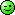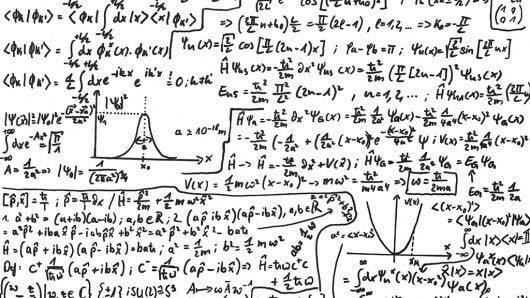Re: Are You Good At Maths? Solve This by hatux: 8:55am On Mar 07, 2015 The first question answer is 12, using BODMAS. We first multiply 1 by 0 which gives us 0 and then add all together, which gives 12. The second question answer is 26, when adding the number following each on the left hand side and the answer you got on the right. 2 + 3 =5 + 5=10, 3+4=7+10=17, 4+5=9+17=26.